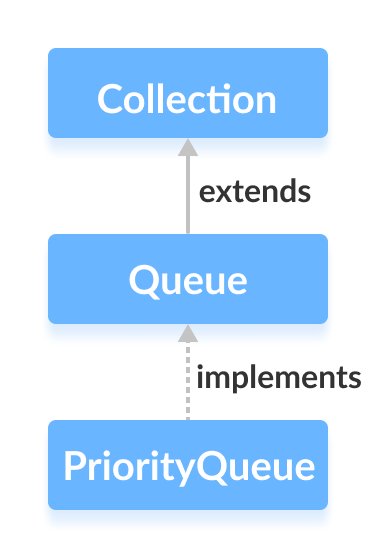# Java PriorityQueue

The `PriorityQueue` class provides the functionality of the heap data structure.

It implements the Queue interface.Unlike normal queues, priority queue elements are retrieved in sorted order.

Suppose, we want to retrieve elements in the ascending order. In this case, the head of the priority queue will be the smallest element. Once this element is retrieved, the next smallest element will be the head of the queue.

It is important to note that the elements of a priority queue may not be sorted. However, elements are always retrieved in sorted order.

## Creating PriorityQueue

In order to create a priority queue, we must import the `java.util.PriorityQueue` package. Once we import the package, here is how we can create a priority queue in Java.

``````PriorityQueue<Integer> numbers = new PriorityQueue<>();
``````

Here, we have created a priority queue without any arguments. In this case, the head of the priority queue is the smallest element of the queue. And elements are removed in ascending order from the queue.

However, we can customize the ordering of elements with the help of the `Comparator` interface. We will learn about that later in this tutorial.

## Methods of PriorityQueue

The `PriorityQueue` class provides the implementation of all the methods present in the `Queue` interface.

## Insert Elements to PriorityQueue

• `add()` - Inserts the specified element to the queue. If the queue is full, it throws an exception.
• `offer()` - Inserts the specified element to the queue. If the queue is full, it returns `false`.

For example,

``````import java.util.PriorityQueue;

class Main {
public static void main(String[] args) {

// Creating a priority queue
PriorityQueue<Integer> numbers = new PriorityQueue<>();

System.out.println("PriorityQueue: " + numbers);

// Using the offer() method
numbers.offer(1);
System.out.println("Updated PriorityQueue: " + numbers);
}
}
``````

Output

```PriorityQueue: [2, 4]
Updated PriorityQueue: [1, 4, 2]
```

Here, we have created a priority queue named numbers. We have inserted 4 and 2 to the queue.

Although 4 is inserted before 2, the head of the queue is 2. It is because the head of the priority queue is the smallest element of the queue.

We have then inserted 1 to the queue. The queue is now rearranged to store the smallest element 1 to the head of the queue.

## Access PriorityQueue Elements

To access elements from a priority queue, we can use the `peek()` method. This method returns the head of the queue. For example,

``````import java.util.PriorityQueue;

class Main {
public static void main(String[] args) {

// Creating a priority queue
PriorityQueue<Integer> numbers = new PriorityQueue<>();
System.out.println("PriorityQueue: " + numbers);

// Using the peek() method
int number = numbers.peek();
System.out.println("Accessed Element: " + number);
}
}
``````

Output

```PriorityQueue: [1, 4, 2]
Accessed Element: 1
```

## Remove PriorityQueue Elements

• `remove()` - removes the specified element from the queue
• `poll()` - returns and removes the head of the queue

For example,

``````import java.util.PriorityQueue;

class Main {
public static void main(String[] args) {

// Creating a priority queue
PriorityQueue<Integer> numbers = new PriorityQueue<>();
System.out.println("PriorityQueue: " + numbers);

// Using the remove() method
boolean result = numbers.remove(2);
System.out.println("Is the element 2 removed? " + result);

// Using the poll() method
int number = numbers.poll();
System.out.println("Removed Element Using poll(): " + number);
}
}
``````

Output

```PriorityQueue: [1, 4, 2]
Is the element 2 removed? true
Removed Element Using poll(): 1
```

## Iterating Over a PriorityQueue

To iterate over the elements of a priority queue, we can use the `iterator()` method. In order to use this method, we must import the `java.util.Iterator` package. For example,

``````import java.util.PriorityQueue;
import java.util.Iterator;

class Main {
public static void main(String[] args) {

// Creating a priority queue
PriorityQueue<Integer> numbers = new PriorityQueue<>();
System.out.print("PriorityQueue using iterator(): ");

//Using the iterator() method
Iterator<Integer> iterate = numbers.iterator();
while(iterate.hasNext()) {
System.out.print(iterate.next());
System.out.print(", ");
}
}
}
``````

Output

```PriorityQueue using iterator(): 1, 4, 2,
```

## Other PriorityQueue Methods

Methods Descriptions
`contains(element)` Searches the priority queue for the specified element. If the element is found, it returns `true`, if not it returns `false`.
`size()` Returns the length of the priority queue.
`toArray()` Converts a priority queue to an array and returns it.

## PriorityQueue Comparator

In all the examples above, priority queue elements are retrieved in the natural order (ascending order). However, we can customize this ordering.

For this, we need to create our own comparator class that implements the `Comparator` interface. For example,

``````import java.util.PriorityQueue;
import java.util.Comparator;
class Main {
public static void main(String[] args) {

// Creating a priority queue
PriorityQueue<Integer> numbers = new PriorityQueue<>(new CustomComparator());
System.out.print("PriorityQueue: " + numbers);
}
}

class CustomComparator implements Comparator<Integer> {

@Override
public int compare(Integer number1, Integer number2) {
int value =  number1.compareTo(number2);
// elements are sorted in reverse order
if (value > 0) {
return -1;
}
else if (value < 0) {
return 1;
}
else {
return 0;
}
}
}
``````

Output

```PriorityQueue: [4, 3, 1, 2]
```

In the above example, we have created a priority queue passing CustomComparator class as an argument.

The CustomComparator class implements the `Comparator` interface.

We then override the `compare()` method. The method now causes the head of the element to be the largest number.